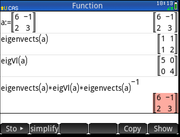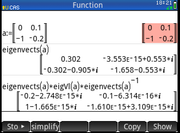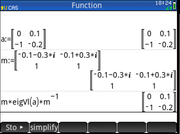eigenvects function returning wrong answers for complex eigenvectors
01-01-2020, 05:47 PM (This post was last modified: 01-01-2020 06:14 PM by medwatt.)
Post: #1
 medwattJunior Member Posts: 23 Joined: Jun 2015
eigenvects function returning wrong answers for complex eigenvectors
I was working on a problem and was getting funny results. I thought there was an issue with my matrices because I didn't expect the calculator to be giving wrong results.

Before, I present the issue, I want to point out that the function works fine for matrices with real eigenvalues/eigenvectors. In the last step, I'm using the matrix diagonalization formula.This is the matrix I'm working on. It has two complex conjugate eigenvalues (and therefore eigenvectors). Because the eigenvector matrix returned is wrong, I wasn't able to get the original matrix using the matrix diagonalization formula, as was the case in the previous example.If I manually enter the correct eigenvectors, I get the original matrix. This proves that the eigenvects function is returning wrong results when the eigenvectors are complex. The correct eigenvectors are shown in the "m" matrix.01-02-2020, 09:05 AM
Post: #2
 parisseSenior Member Posts: 1,051 Joined: Dec 2013
RE: eigenvects function returning wrong answers for complex eigenvectors
The reason is that eigVl and eigenvects do not necessarily sort the eigenvectors the same way. You should use jordan to compute both simultaneously:
a:=[[0,0.1],[-1,-0.2]];
p,d:=jordan(a);
p*d*p^-1;
In addition, this is more efficient (for large matrices).
01-02-2020, 11:14 AM (This post was last modified: 01-02-2020 11:16 AM by medwatt.)
Post: #3
 medwattJunior Member Posts: 23 Joined: Jun 2015
RE: eigenvects function returning wrong answers for complex eigenvectors
(01-02-2020 09:05 AM)parisse Wrote:  The reason is that eigVl and eigenvects do not necessarily sort the eigenvectors the same way. You should use jordan to compute both simultaneously:
a:=[[0,0.1],[-1,-0.2]];
p,d:=jordan(a);
p*d*p^-1;
In addition, this is more efficient (for large matrices).

I'm actually not interested in using the diagonalization formula. What I'm interested in is the result from the eigenvects function. Why doesn't the function return a simple answer?

[[-0.1-0.3*i, -0.1+0.3*i],[1,1]] - this is the simple answer I expect. Instead, what I got is something that doesn't even tell me that the eigenvectors are complex conjugates. I've tried it on Wolfram alpha and lots of other websites and all of them return [[-0.1-0.3*i, -0.1+0.3*i],[1,1]].
01-02-2020, 01:42 PM
Post: #4
 WernerSenior Member Posts: 375 Joined: Dec 2013
RE: eigenvects function returning wrong answers for complex eigenvectors
In this case, I agree. The matrix is 2x2 real, and has complex conjugate eigenvalues and thus complex conjugate eigenvectors. The 48GX series gets it right.

Cheers, Werner
01-03-2020, 03:25 PM
Post: #5
 parisseSenior Member Posts: 1,051 Joined: Dec 2013
RE: eigenvects function returning wrong answers for complex eigenvectors
The algorithm I'm using for diagonalization is a generic algorithm that does not check for real matrix/complex conjugate answers. I'd like to point that *the answer is perfectly correct, not wrong* : you can multiply an eigenvector by any non-zero constant and you will still get an eigenvector, the title of this thread is therefore misleading.
Remarks:
1/ for approx matrices, the expected normalization of eigenvectors is probably a vector of norm 1. For exact matrices, I don't think there is a convention.
2/ eigenvects(exact(a)) or jordan(exact(a)) returns simple answers, and you can of course replace in this answer an eigenvector by the conjugate eigenvector of the conjugate eigenvalue.
01-03-2020, 04:05 PM
Post: #6
 medwattJunior Member Posts: 23 Joined: Jun 2015
RE: eigenvects function returning wrong answers for complex eigenvectors
(01-03-2020 03:25 PM)parisse Wrote:  1/ for approx matrices, the expected normalization of eigenvectors is probably a vector of norm 1. For exact matrices, I don't think there is a convention.
2/ eigenvects(exact(a)) or jordan(exact(a)) returns simple answers, and you can of course replace in this answer an eigenvector by the conjugate eigenvector of the conjugate eigenvalue.

These are better. I never knew that it was convention to normalize the last row of a the eigenvector matrix to 1 if the results were approximate. Maybe, I'll write a small routine to take care of that.
 « Next Oldest | Next Newest »

User(s) browsing this thread: 1 Guest(s)